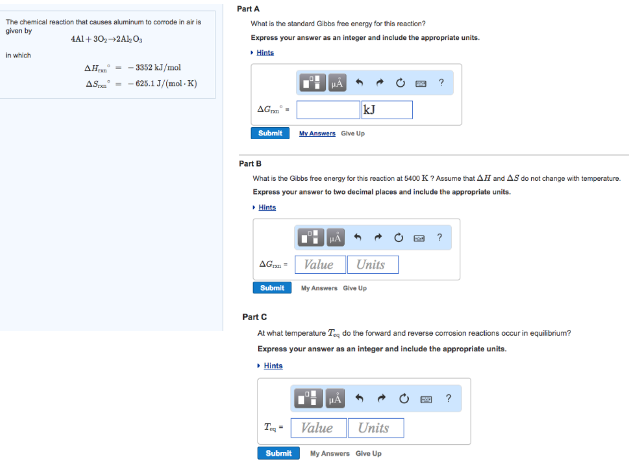# Problem: The chemical reaction that causes aluminum to corrode in air is given by 4Al + 3O2 → 2Al2O3Part AWhat is the standard Gibbs free energy for this reaction? Express your answer as an integer and include the appropriate units. Part BWhat is the Gibbs free energy for this reaction at 5400 K? Assume that ΔH and ΔS do not change with temperature. Express your answer to two decimal places and include the appropriate units. Part CAt what temperature Teq do the forward and reverse corrosion reaction occur in equilibrium?Express your answer as an integer and include the appropriate units.

###### FREE Expert Solution
88% (440 ratings)###### Problem Details

The chemical reaction that causes aluminum to corrode in air is given by

4Al + 3O2 → 2Al2O3

Part A

What is the standard Gibbs free energy for this reaction?

Part B

What is the Gibbs free energy for this reaction at 5400 K? Assume that ΔH and ΔS do not change with temperature.

Express your answer to two decimal places and include the appropriate units.

Part C

At what temperature Teq do the forward and reverse corrosion reaction occur in equilibrium?